# How to calculate Area Under Curve and Concordant

This tutorial provides detailed explanation and multiple methods to calculate area under ROC curve (AUC), concordant and discordant along with implementation in SAS and R. By default, every statistical packages like SAS, SPSS and R generates these model fit measures when you run syntax for logistic regression. However, it is important to know how these model performance metrics are calculated mathematically. One more reason to know the calculation behind these metrics is it would give you confidence to explain these metrics and you will have an edge over your peers when your predictive model demands calibration or refitting.Understanding Concordant and AUC
Importance of Area under Curve and Concordant
Area under Curve (AUC) or Receiver operating characteristic (ROC) curve is used to evaluate and compare the performance of binary classification model. It measures discrimination power of your predictive classification model. In simple words, it checks how well model is able to distinguish (separates) events and non-events. Suppose you are building a predictive model for bank to identify customers who are likely to buy credit card. In this case case, purchase of credit card is event (or desired outcome) and non-purchase of credit card is non-event.
AUC or ROC curve is a plot of the proportion of true positives (events predicted to be events) versus the proportion of false positives (nonevents predicted to be events). True Positive Rate is also called Sensitivity. False Positive Rate is also called (1-Specificity). Sensitivity is on Y-axis and (1-Specificity) is on X-axis. Higher the AUC score, better the model.

Diagonal line represents random classification model. It is equivalent to prediction by tossing a coin. All points along the diagonal line say same true positive and false positive rate.ROC Curve

Methods to calculate ROC and Concordant

## Manual Calculation to estimate ROC, Concordant, Discordant, Gini

1. Calculate the predicted probability in logistic regression model. It can be any binary classification model, not restricted to logistic regression.
2. Divide the data into two datasets. One dataset contains observations having actual value of dependent variable with value 1 (i.e. event) and corresponding predicted probability values. And the other dataset contains observations having actual value of dependent variable 0 (non-event) against their predicted probability scores.
3. Compare each predicted value in first dataset with each predicted value in second dataset.
4. Total Number of pairs to compare = `x` * `y`
`x` : Number of observations in first dataset (actual values of 1 in dependent variable)
`y` : Number of observations in second dataset (actual values of 0 in dependent variable).

In this step, we are performing cartesian product (cross join) of events and non-events. For example, you have 100 events and 1000 non-events. It would create 100k (100*1000) pairs for comparison.
5. A pair is concordant if 1 (observation with the desired outcome i.e. event) has a higher predicted probability than 0 (observation without the outcome i.e. non-event).
6. A pair is discordant if 0 (observation without the desired outcome i.e. non-event) has a higher predicted probability than 1 (observation with the outcome i.e. event).
7. A pair is tied if 1 (observation with the desired outcome i.e. event) has same predicted probability than 0 (observation without the outcome i.e. non-event).
8. The final percent values are calculated using the formula below -

9. `Percent Concordant = 100*[(Number of concordant pairs)/Total number of pairs]`
`Percent Discordant = 100*[(Number of discordant pairs)/Total number of pairs]`
`Percent Tied = 100*[(Number of tied pairs)/Total number of pairs]`
`Area under curve (c statistics) = (Percent Concordant + 0.5 * Percent Tied)/100`

Interpretation of Concordant, Discordant and Tied Percent

Percent Concordant : Percentage of pairs where the observation with the desired outcome (event) has a higher predicted probability than the observation without the outcome (non-event).

Percent Discordant : Percentage of pairs where the observation with the desired outcome (event) has a lower predicted probability than the observation without the outcome (non-event).

Percent Tied : Percentage of pairs where the observation with the desired outcome (event) has same predicted probability than the observation without the outcome (non-event).

c statistics (AUC) : c-statistics is also called area under curve (AUC). Some statisticians also call it AUROC which stands for area under the receiver operating characteristics. It is calculated by adding Concordance Percent and 0.5 times of Tied Percent.

Gini coefficient or Somers' D statistic is closely related to AUC. It is calculated by (2*AUC - 1).

In general, higher percentages of concordant pairs and lower percentages of discordant and tied pairs indicate a more desirable model.

SAS and R Code for ROC, Concordant / Discordant :
Download the CSV data file from UCLA website.
The code below calculates these performance metrics in SAS and R. It executes each step explained above theoretically.

###### SAS Code
```FILENAME PROBLY TEMP;
PROC HTTP
URL="https://stats.idre.ucla.edu/stat/data/binary.csv"
METHOD="GET"
OUT=PROBLY;
RUN;

OPTIONS VALIDVARNAME=ANY;
PROC IMPORT
FILE=PROBLY
OUT=WORK.binary REPLACE
DBMS=CSV;
RUN;

ods graphics on;
Proc logistic data= WORK.binary descending plots(only)=roc;
class rank / param=ref ;
model admit = gre gpa rank;
output out = estprob p= pred;
run;

/*split the data into two datasets- event and non-event*/
Data event nonevent;
Set estprob;
If admit = 1 then output event;
else if admit = 0 then output nonevent;
run;

/*Cartesian product of event and non-event actual cases*/
Proc SQL noprint;
create table pairs as
select a.admit as admit1, b.admit as admit0,
a.pred as pred1,b.pred as pred0
from event a cross join nonevent b;
quit;

/*Calculating concordant,discordant and tied percent*/
Data pairs;
set pairs;
concordant =0;
discordant=0;
tied=0;
If pred1 > pred0 then concordant = 1;
else If pred1 < pred0 then discordant = 1;
else tied = 1;
run;

/*Mean values - Final Result*/
proc sql;
select mean(Concordant)*100 as Percent_Concordant,
mean(Discordant) *100 as Percent_Discordant,
mean(Tied)*100 as Percent_Tied,
(calculated Percent_Concordant + 0.5* calculated Percent_Tied)/100 as AUC,
2*calculated AUC - 1 as somers_d
from pairs;
quit;
```
###### R Code
```# Read Data
df = read.csv("https://stats.idre.ucla.edu/stat/data/binary.csv")

# Factor Variables
df\$admit = as.factor(df\$admit)
df\$rank = as.factor(df\$rank)

# Logistic Model
df\$rank <- relevel(df\$rank, ref='4')
mylogistic <- glm(admit ~ ., data = df, family = "binomial")
summary(mylogistic)\$coefficient

# Predict
pred = predict(mylogistic, type = "response")
finaldata = cbind(df, pred)

AUC <- function (actuals, predictedScores){
fitted <- data.frame (Actuals=actuals, PredictedScores=predictedScores)
colnames(fitted) <- c('Actuals','PredictedScores')
ones <- fitted[fitted\$Actuals==1, ] # Subset ones
zeros <- fitted[fitted\$Actuals==0, ] # Subsetzeros
totalPairs <- nrow (ones) * nrow (zeros) # calculate total number of pairs to check
conc <- sum (c(vapply(ones\$PredictedScores, function(x) {((x > zeros\$PredictedScores))}, FUN.VALUE=logical(nrow(zeros)))), na.rm=T)
disc <- sum(c(vapply(ones\$PredictedScores, function(x) {((x < zeros\$PredictedScores))}, FUN.VALUE = logical(nrow(zeros)))), na.rm = T)
concordance <- conc/totalPairs
discordance <- disc/totalPairs
tiesPercent <- (1-concordance-discordance)
AUC = concordance + 0.5*tiesPercent
Gini = 2*AUC - 1
return(list("Concordance"=concordance, "Discordance"=discordance,
"Tied"=tiesPercent, "AUC"=AUC, "Gini or Somers D"=Gini))
}

AUC(finaldata\$admit, finaldata\$pred)
```Result

## Using Integration to calculate ROC, Gini

Trapezoidal Rule Numerical Integration method is used to find area under curve. The area of a trapezoid is
( xi+1 – xi ) * ( yi + yi+1 ) / 2
In our case, x refers to values of false positive rate (1-Specificity) at different probability cut-offs, y refers to true positive rate (Sensitivity) at different cut-offs. Vector x needs to be sorted. Any observation with predicted probability that exceeds or equals probability cut-off is predicted to be an event; otherwise, it is predicted to be a nonevent.
( fpri+1 – fpri ) * ( tpri + tpri+1 ) / 2
`fpr` represents false positive rate (1- specificity). `tpr` represents true positive rate (sensitivity). See the image below showing step by step calculation. It includes a very few cut-offs for demonstration purpose.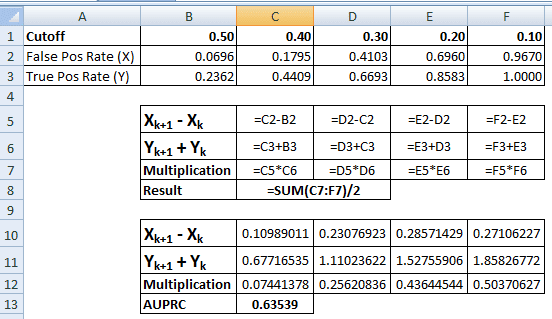Integration Calculation

###### SAS Code
In the SAS program below, we are using `PROC IML` procedure to perform integration calculations.
```FILENAME PROBLY TEMP;
PROC HTTP
URL="https://stats.idre.ucla.edu/stat/data/binary.csv"
METHOD="GET"
OUT=PROBLY;
RUN;

OPTIONS VALIDVARNAME=ANY;
PROC IMPORT
FILE=PROBLY
OUT=WORK.binary REPLACE
DBMS=CSV;
RUN;

ods graphics on;
Proc logistic data= WORK.binary descending plots(only)=roc;
class rank / param=ref ;
model admit = gre gpa rank / outroc=performance;
output out = estprob p= pred;
run;

proc sort data=performance;
by _1MSPEC_;
run;

proc iml;
use performance;
read all var {_SENSIT_} into sensitivity;
read all var {_1MSPEC_} into falseposrate;
N  = 2 : nrow(falseposrate);
fpr = falseposrate[N] - falseposrate[N-1];
tpr = sensitivity[N] + sensitivity[N-1];
ROC = fpr`*tpr/2;
Gini= 2*ROC - 1;
print ROC Gini;
```
###### R Code
```# Read Data
df = read.csv("https://stats.idre.ucla.edu/stat/data/binary.csv")

# Factor Variables
df\$admit = as.factor(df\$admit)
df\$rank = as.factor(df\$rank)

# Logistic Model
df\$rank <- relevel(df\$rank, ref='4')
mylogistic <- glm(admit ~ ., data = df, family = "binomial")
summary(mylogistic)\$coefficient

# Predict
pred = predict(mylogistic, type = "response")
finaldata = cbind(df, pred)

library(ROCR)
predobj <- prediction(finaldata\$pred, finaldata\$admit)
perf <- performance(predobj,"tpr","fpr")
plot(perf)

# Trapezoidal rule of integration
# Computes the integral of Sensitivity (Y) with respect to FalsePosRate (x)
x = perf@x.values[]
y = perf@y.values[]
idx = 2:length(x)
testdf=data.frame(FalsePosRate = (x[idx] - x[idx-1]), Sensitivity = (y[idx] + y[idx-1]))
(AUROC = sum(testdf\$FalsePosRate * testdf\$Sensitivity)/2)
```Related Posts
About Author:Deepanshu founded ListenData with a simple objective - Make analytics easy to understand and follow. He has over 8 years of experience in data science. During his tenure, he has worked with global clients in various domains like Banking, Insurance, Telecom and Human Resource.

18 Responses to "How to calculate Area Under Curve and Concordant"
1.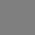Very precise and clear explanation of concordance and discordance. Also the code helps in better understanding of the phenomenon. Thanks.

1.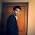Thank you for your appreciation. Cheers!

2.Neat explanations, really helpful to understood these definitions. Thanks!

3.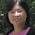Very clear explanation, thank you :)

4.Thanks for the post! Shouldn't it be proc logistic with descending option? as we are treating 1s as events and 0 as nonevents

1.Corrected! Thanks for pointing it out.

5.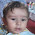First time I understood concordance and discordance. Thanks

6.For a good model what should be the concordance?

1.Concordance Percent should be 80 or above.

7.Very good explanation

8.Very informative, clear, and to the point

9.Very good explanation and informative. Thanks Buddy keep sharing

10.Can you please give the calculation of concordance and disconcordance in excel format with example which will be easy to understand the calculation.

11.The above codes are very useful. Any suggestions for weighted data?

12.Hello, I want to know, what to do in cases where tied percentage is high, say 20%. How to reduce tied percentage?

13.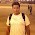Excellent Work. Thanks for such detailed description.

14.Not as clear as needed

15.Next → ← Prev
Love this Post? Spread the Word!
Share